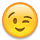If you have a new account but are having problems posting or verifying your account, please email us on [email protected] for help. Thanks :)

# Numbers Puzzles

• 06-02-2009 5:32pm

Using five 2s and as many plus symbols as you need, make an equation that equals 28

22 + 2 + 2 + 2 = 28

Another one, now that you see how they work.

Using eight 8s and as many plus symbols as you need, make an equation that equals 1000

888 + 88 + 8 + 8 + 8 = 1000

• Using five 2s and as many plus symbols as you need, make an equation that equals 28

22 + 2 + 2 + 2 = 28

Another one, now that you see how they work.

Using eight 8s and as many plus symbols as you need, make an equation that equals 1000

very good, but a bit complicated at the start